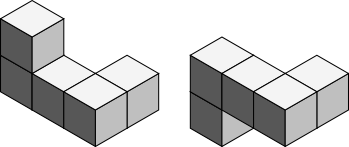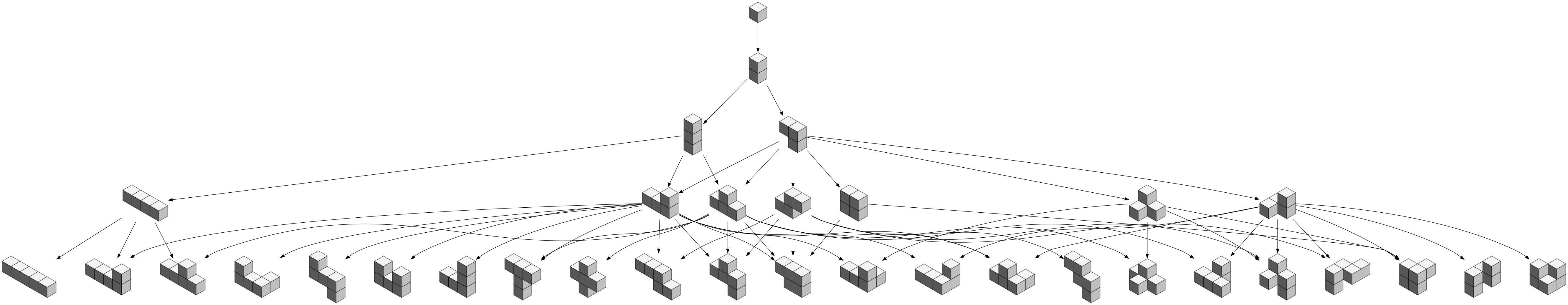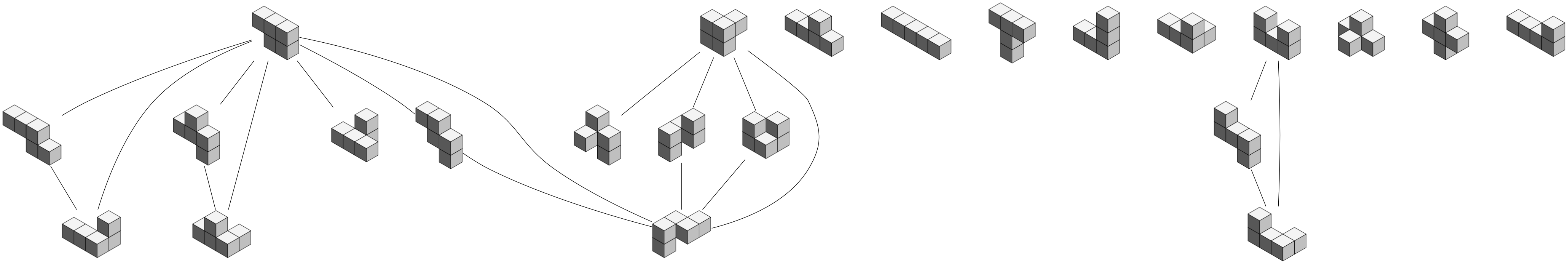#tianjara.net |Andrew Harvey's Blog

Polyhypercubes and That Whole Area of Combinatorics...
4th February 2012

I've pulled this post from my draft archives from July 2010. I think it is about time I post it.

I stumbled upon Tetris the other day and began to wonder about the pieces, which lead me to stumble into a whole area of maths that I didn't know had been documented: polyforms.

In 2D you have n squares which can be formed into Polyominos, for an arrangement of squares to form a polyomino each square must share at least one of it's edges with another square. If you use 2 squares you get Dominos, 3 squares gives you Trominos, 4 gives Tetrominos, 5 gives Pentominos, and so on to n-minos. These all fall under the category of polyminos.

In 3D you have cubes with the principle that each cube must share a face with at least one other cube. These shapes are called polycubes. If you use 3 cubes you get tricubes, and so on.

You can extrapolate this concept to nD hypercubes with the principle that each n-cube must share at least one of its (n-1)-faces with another n-cube. Lets call these polyhypercubes.

We shall also say that polyhypercubes are the same if we can rotate (or mirror as well, depending on your definition of equality) them to match exactly. Note that if you are looking at the set of all poly-n-cubes for some n, the total size will vary depending whether you decide to allow mirroring (...although mirroring is the same as allowing rotation in a dimension 1 higher than the dimension of the space).Two pentacubes which are the same if you count mirroring as an allowed operation when testing for equality, but not the same if you don't (you can't rotate one to fall on the other)."

To generate these shapes you can start with one cube, from this you can make a graph where you add one block to every possible place. You can turn this into a directed graph, where an edge indicates you can get one shape by adding another cube to the previous shape. Perhaps this can be extended to a hypergraph, where two shapes are linked if you can morph from one to another by moving just one block (square, cube...)?

[caption id="attachment_1381" align="aligncenter" width="1024" caption="Evolution of the 5-polycubes where an edge represents adding one cube to a polycube."][/caption]

You can also do a similar graph (well actually it would be a multigraph as you would have islands) by only allowing an edge where you can make a "rubix cube" like change to the object.

[caption id="attachment_1382" align="aligncenter" width="1024" caption="All 5-polycubes where an edge indicates a "rubix cube" like transformation."][/caption]

I was originally interested in two problems here. Generating all possible n-polycubes for a given n, and finding the total number of n-polycubes for a given n. The diagrams are examples of what I mean, but it was only done manually and only up to 5-polycubes. Code used to generate those diagrams is at https://github.com/andrewharvey/phc/tree/master/concrete-cases/5-polycubes.

Tags: mathematics.